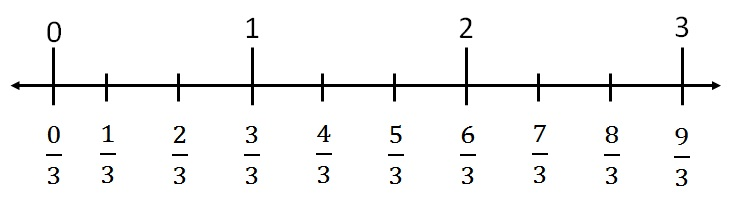Comparing fractions

Chapter 7 Class 6 Fractions
Concept wise

Like fractions are those fractions where denominators are same

Eg : 1/3, 2/3, 4/3, 8/3 are like fractions

How do we know which one is bigger?

The fraction where numerator is greater, that fraction is bigger

1/3 < 2/3 < 4/3 < 8/3Learn in your speed, with individual attention - Teachoo Maths 1-on-1 Class

### Transcript

Comparing like fractionsLike fractions are those fractions where denominators are same Eg : 1/3, 2/3, 4/3, 8/3 are like fractions How do we know which one is bigger? The fraction where numerator is greater, that fraction is bigger 1/3 < 2/3 < 4/3 < 8/3